Get inspired by the success stories of our students in IIT JAM MS, ISI  MStat, CMI MSc DS.  Learn More

# Venny Venny AMy GMy | ISI MStat 2016 PSB Problem 3This problem is a very basic and cute application of set theory, Venn diagram and AM GM inequality to solve the ISI MStat 2016 PSB Problem 3.

## Problem - Venn diagram and AM GM inequality

For any two events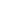and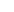, show that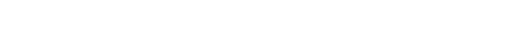## Solution

#### Draw the Venn Diagram

P(region Red) =P(region Blue) =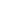P(region Grey) =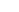P(region Brown) =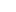Observe that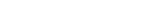.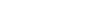.

#### Now, Calculate Given Probability of Sets in terms of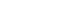.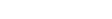.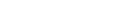.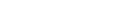.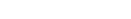.

#### The Final Inequality..

Observe that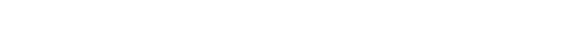.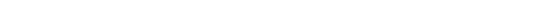by AM - GM Inequality.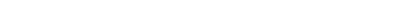.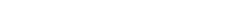.

Hence,This problem is a very basic and cute application of set theory, Venn diagram and AM GM inequality to solve the ISI MStat 2016 PSB Problem 3.

## Problem - Venn diagram and AM GM inequality

For any two eventsand, show that## Solution

#### Draw the Venn Diagram

P(region Red) =P(region Blue) =P(region Grey) =P(region Brown) =Observe that..

#### Now, Calculate Given Probability of Sets in terms of.....

#### The Final Inequality..

Observe that.by AM - GM Inequality...

Hence,This site uses Akismet to reduce spam. Learn how your comment data is processed.

### Knowledge Partner